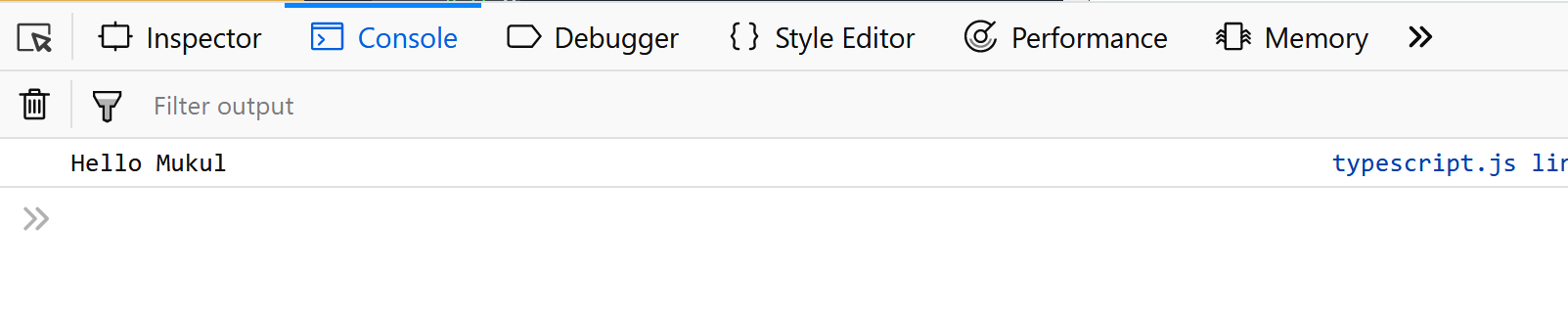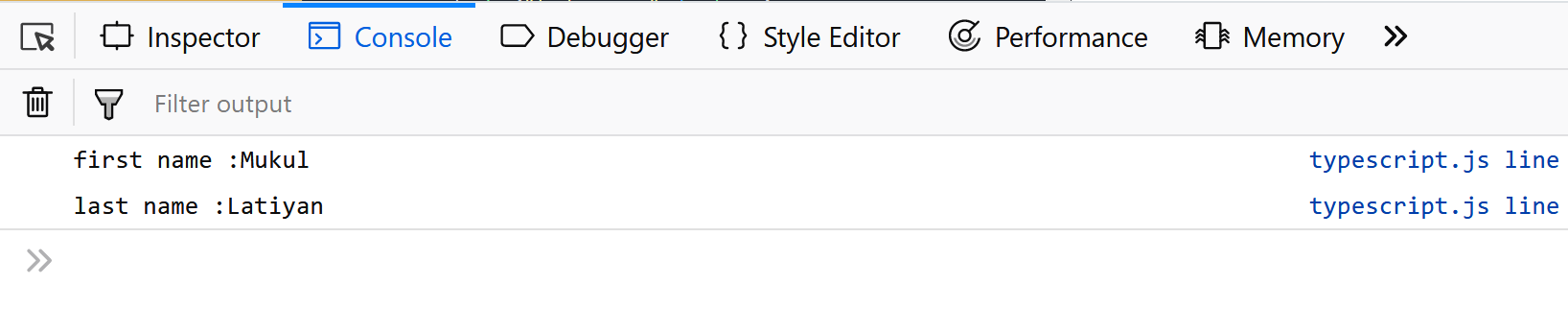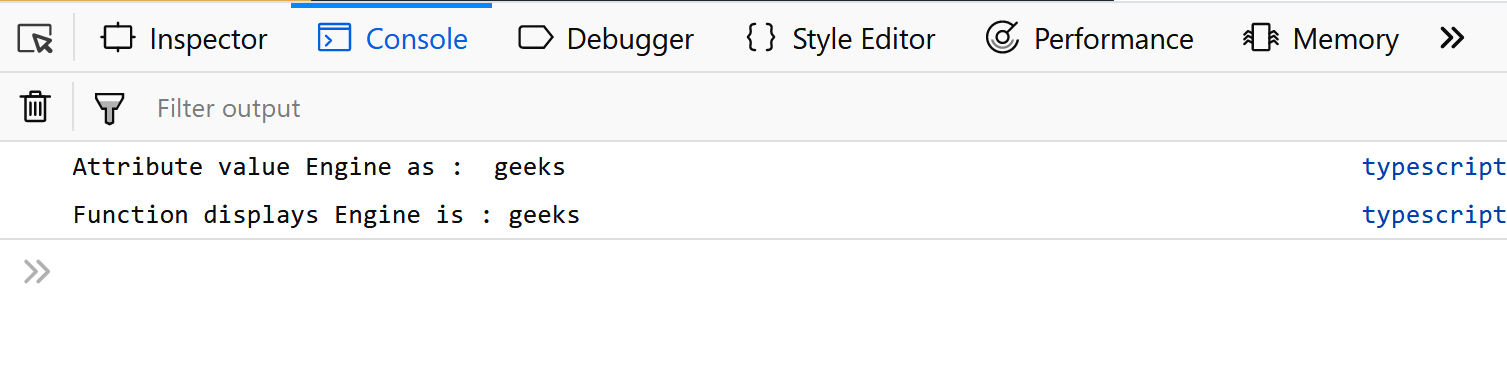GeeksforGeeks App
Open AppBrowser
Continue

## Related Articles

In terms of OOPs(Object Oriented Programming), a class is a blueprint which is used for creating objects. A class is a collection of objects having common properties.It contains methods,constructors,blocks,nested classes,interfaces etc. Objects are basically the entities that have some properties like an object in the real world(chair, table etc). Typescript is an open source programming language which is built over Javascript, also known as Superset of Javascript. Typescript has more features as when compared to the Javascript. It supports Object Oriented programming features like classes, Interface, Polymorphism etc.

Syntax to declare a class:

## javascript

 `// typescript code``class class_name{``    ``field;``    ``method;``}`

The above code when passed into a typescript compiler will get converted into the javascript code shown below. We are free to use any name instead of class_name.

## javascript

 `// code converted to javascript``var` `class_name = ``/** @class */` `(``function` `() {``    ``function` `class_name() {``    ``}``    ``return` `class_name;``}());`

Note: The typescript code is saved using the .ts extension. Let’s see some typescript examples too:

## javascript

 `// typescript code``class Student {``    ``studCode: number;``    ``studName: string;`` ` `    ``constructor(code: number, name: string) {``        ``this``.studName = name;``        ``this``.studCode = code;``    ``}``    ``getGrade() : string {``        ``return` `"A+"` `;``     ``}``} `

The example declare a Student class which has two fields that is studCode and studName and a constructor which is special type of function which is responsible for variable or object initialization. Here it is parameterized constructor(already having the parameters). And this keyword which refers to the current instance of the class. getGrade() is a simple function which returns a string. The above typescript code will be converted into the javascript code as shown below:

## javascript

 `// converted javascript code``var` `Student = ``/** @class */` `(``function` `() {``    ``function` `Student(code, name) {``        ``this``.studName = name;``        ``this``.studCode = code;``    ``}``    ``Student.prototype.getGrade = ``function` `() {``        ``return` `"A+"``;``    ``};``    ``return` `Student;``}());`

Objects An object is an instance of class which contains set of key value pairs. It’s value may be scalar values or functions or even array of other objects.

Syntax of an Object looks like the code below:

## javascript

 `// simple object code in javascript``let object_name = { ``   ``key1: “value”,  ``   ``key2: ``function``() {``      ``//functions ``   ``}, ``   ``key3:[“content1”, “content2”] ``//collection  ``};`

An object can contain scalar value, functions and structures like arrays and tuples. Let’s see with simple example:

## javascript

 `// simple javascript code``let person = {``   ``fName:``"Mukul"``, ``   ``lName:``"Latiyan"``, ``   ``Hello:``function``() {  }  ``//Type template ``} ``person.Hello = ``function``() {  ``   ``console.log(``"Hello "``+person.fName)``}  ``person.Hello()`

Output:## javascript

 `// typescript object example``var` `person = { ``   ``fname:``"Mukul"``, ``   ``lname:``"Latiyan"` `}; ``var` `hello = ``function``(obj: { fname:string, lname :string }) { ``   ``console.log(``"first name :"``+obj.fname) ``   ``console.log(``"last name :"``+obj.lname) ``} ``hello(person)`

Output:For creating Instance Objects. To create an instance of the class, use with the new keyword followed by the class name. To allocates memory for objects with the help new during runtime. Like:

## javascript

 `let object_name = ``new` `class_name([ arguments ])`

In order to create an instance of an object we can do something like the code below.

## javascript

 `let obj = ``new` `Student();  `

Accessing Attributes and Functions: A class’s attributes and functions can be accessed by the object. With the help of ‘ . ’ dot notation or bracket notation([”]) we access the data members of a class.

```//accessing an attribute
obj.field_name or obj['field_name']

//accessing a function
obj.function_name()```

Consider the code below:

## javascript

 `// typescript code``class Car { ``   ``//field ``   ``engine:string; ``    ` `   ``//constructor ``   ``constructor(engine:string) { ``      ``this``.engine = engine ``   ``}  ``    ` `   ``//function ``   ``display():void { ``      ``console.log(``"Function displays Engine is  :   "``+``this``.engine) ``   ``} ``} `` ` `//create an object ``var` `o1 = ``new` `Car(``"geeks"``)`` ` `//access the field ``console.log(``"Reading attribute value Engine as :  "``+o1.engine)  `` ` `//access the function``o1.display()`

After compilation this code will be converted into the javascript shown below:

## javascript

 `// converted javascript code``var` `Car = ``/** @class */` `(``function` `() {``    ``//constructor ``    ``function` `Car(engine) {``        ``this``.engine = engine;``    ``}``    ``//function ``    ``Car.prototype.display = ``function` `() {``        ``console.log(``"Function displays Engine is  :   "` `+ ``this``.engine);``    ``};``    ``return` `Car;``}());``//create an object ``var` `o1 = ``new` `Car(``"geeks"``);``//access the field ``console.log(``"Reading attribute value Engine as :  "` `+ o1.engine);``//access the function``o1.display();`

Output:My Personal Notes arrow_drop_up
Related Tutorials